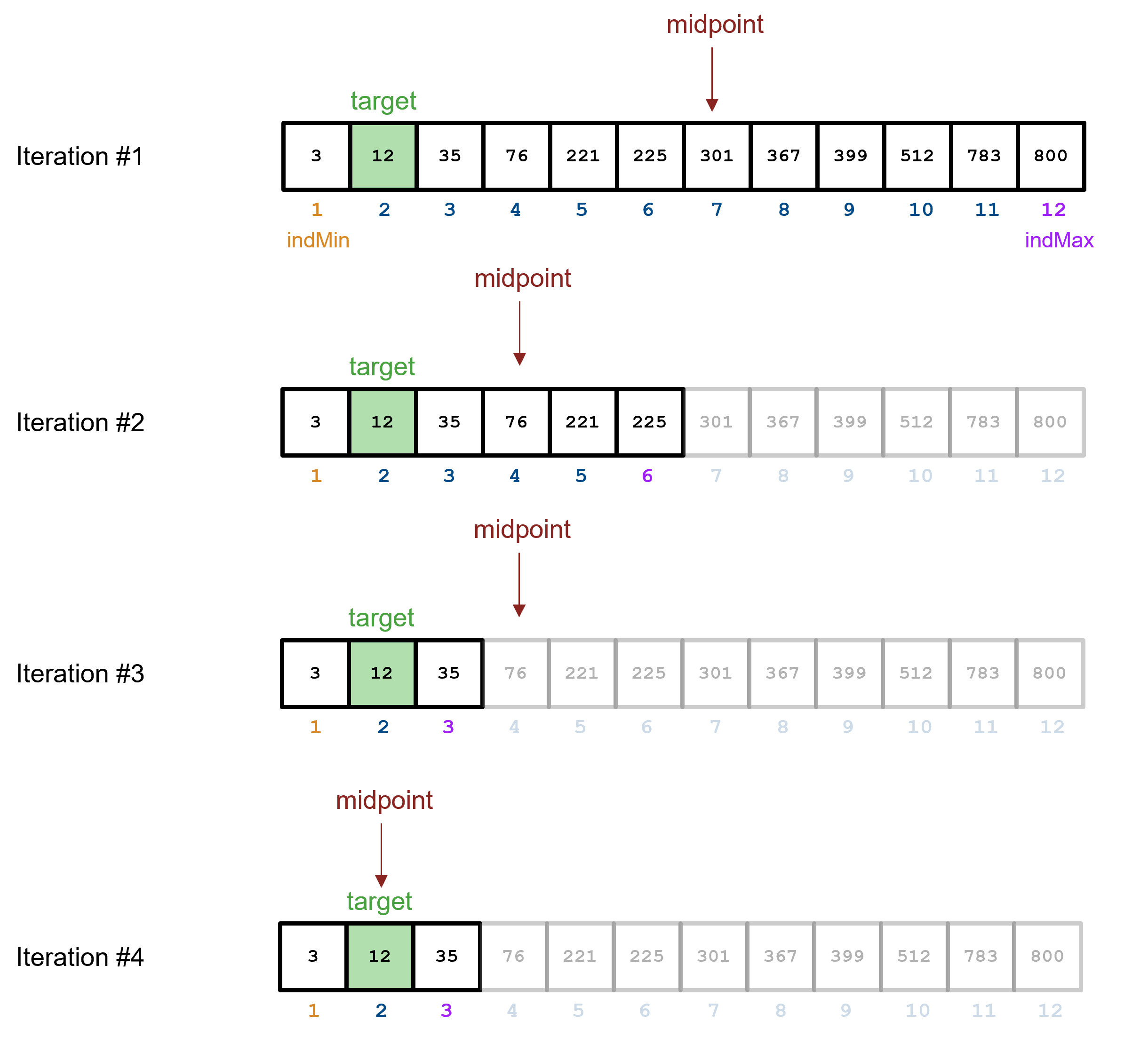# Problem 56040. A Binary Search

One way to locate a target value in a sorted array, is to use a binary search algorithm. Here, you test if the midpoint in the array is the target value. If it is, great! You're done. If not, then you continually narrow your search area depending on whether the target is less than or greater than the midpoint. The algorithm is as follows:
Given an array of sorted values (X), and a target value you wish to locate (target)
1. Calculate the index of the midpoint of the array X by taking the average of the largest and smallest indices and rounding to the nearest integer.
2. If the value located at the midpoint matches your target, set found to true.
3. If the target is less than the value located at the midpoint of X, narrow your search to the lower half of X by setting the largest index in the array to the midpoint - 1.
4. If the target is greater than the value located at the midpoint of X, narrow your search to the upper half of X by setting the smallest index in the array to the midpoint + 1.
5. Repeat the steps above until found is true.Write a function that takes X and target as inputs, performs a binary search and outputs the index of target value as well as the number of iterations it took to find the target.

### Solution Stats

19.02% Correct | 80.98% Incorrect
Last Solution submitted on Nov 22, 2023

### Community Treasure Hunt

Find the treasures in MATLAB Central and discover how the community can help you!

Start Hunting!